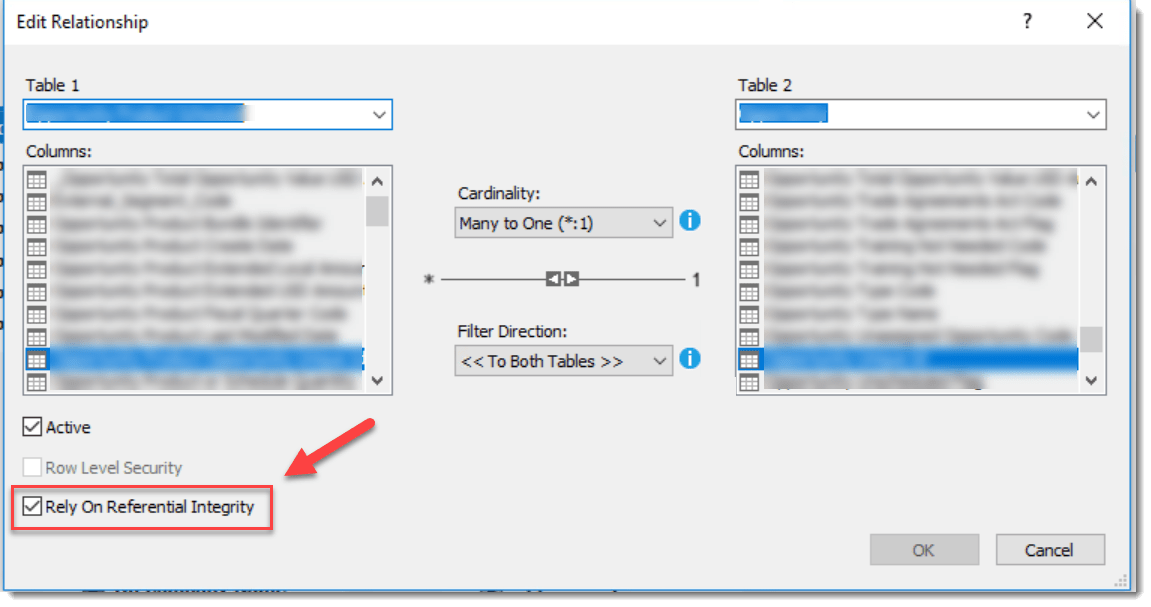# Tip to speed up Direct Query

This is not so much a DAX tip, rather something you might consider if you want to speed up the performance of any Direct Query (DQ) model you manage in Power BI or Analysis Services.

The simple tip is to set relationships to use INNER JOIN rather than LEFT OUTER JOIN in the model. Once this property is set on relationships, your source system should have less work to do to satisfy each DQ queries involving calculations that run across tables, and return faster.

### To set in Power BI Desktop

To find the property in Power BI Desktop, click a relationship in the model view and check the box next to Assume referential Integrity as shown.

A warning is displayed, but click OK.Setting the Assume referential Integrity property on a relationship in Power BI Desktop.
PropertyStatusJoin Type Used
Assume referential Integrity CheckedINNER JOIN
Assume referential Integrity Not CheckedLEFT JOIN

### To Set in Analysis Services

To make the same change in an Analysis Services model, click a relationship in the diagram view and check the Rely on Referential Integrity checkbox as shown,Setting the Rely on Referential Integrity property on a relationship in Analysis Services.
PropertyStatusJoin Type Used
Rely on Referential Integrity CheckedINNER JOIN
Rely on Referential Integrity Not CheckedLEFT JOIN

### The effect

The effect of setting this property means T-SQL generated by Analysis Services uses an INNER JOIN between the tables instead of a LEFT OUTER JOIN.

When LEFT OUTER JOIN gets used, values in rows from the FACT table can be considered by measures despite having no corresponding row in a DIMENSION table.

When an INNER JOIN gets used, only values in rows from the FACT table that have a related value in a DIMENSION table get considered. If the two tables involved in the query are indexed appropriately, the query engine inside the source system can produce the result more efficiently.

Consider the following simple two-table DQ data set – the FACT.Sales table has 6 rows joined to the DIM.Category table using the CategoryID column on both sides.

If the property gets set, so a LEFT OUTER JOIN gets used, any visual that uses a measure that sums the QTY column in FACT sales along with the Category column from the DIM table on the axis can show the Qty amounts associated with CategoryID C; however, these appear as (Blank).

Whereas, when the same query is run using the setting set to INNER JOIN, the same visual shows as follows:

As you can see, the code for the INNER JOIN does not consider rows in the FACT table that have no corresponding row in the DIM table, so are removed from the results.

#### T-SQL generated with property unchecked

```SELECT
TOP (1000001) [t0].[categoryID],SUM(
CAST([t1].[quantity] as BIGINT)
)
AS [a0]
FROM
((select [\$Table].[categoryID] as [categoryID],
[\$Table].[quantity] as [quantity]
from [dbo].[sales] as [\$Table]) AS [t1]

left outer join

(select [\$Table].[categoryID] as [categoryID]
from [dbo].[category] as [\$Table]) AS [t0] on
(
[t1].[categoryID] = [t0].[categoryID]
)
)

GROUP BY [t0].[categoryID]

```

#### T-SQL generated with property checked

```SELECT
TOP (1000001) [t0].[categoryID],SUM(
CAST([t1].[quantity] as BIGINT)
)
AS [a0]
FROM
((select [\$Table].[categoryID] as [categoryID],
[\$Table].[quantity] as [quantity]
from [dbo].[sales] as [\$Table]) AS [t1]

inner join

(select [\$Table].[categoryID] as [categoryID]
from [dbo].[category] as [\$Table]) AS [t0] on
(
[t1].[categoryID] = [t0].[categoryID]
)
)

GROUP BY [t0].[categoryID]
```

### Summary

If the data source used for the model is clean and in good shape, converting relationships to INNER JOIN should not effect numbers in your report. Otherwise consider which is more important, the performance gain offered by using INNER JOIN versus any reporting need to show values for orphaned rows in your FACT table.

Article Rating
Categories:
Inline Feedbacks